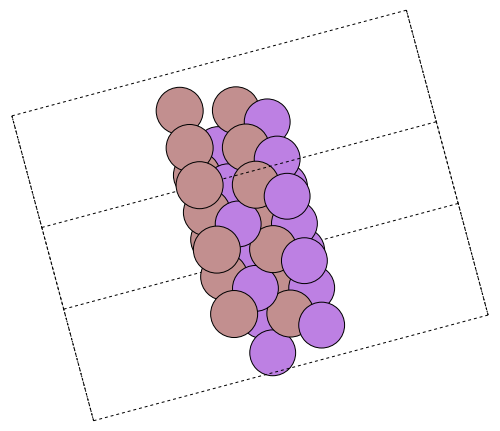# Modelling a gallium arsenide surface

This example shows how to use the atomistic simulation environment, or ASE for short, to set up and run a particular calculation of a gallium arsenide surface. ASE is a Python package to simplify the process of setting up, running and analysing results from atomistic simulations across different simulation codes. By means of ASEconvert it is seamlessly integrated with the AtomsBase ecosystem and thus available to DFTK via our own AtomsBase integration.

In this example we will consider modelling the (1, 1, 0) GaAs surface separated by vacuum.

Parameters of the calculation. Since this surface is far from easy to converge, we made the problem simpler by choosing a smaller Ecut and smaller values for n_GaAs and n_vacuum. More interesting settings are Ecut = 15 and n_GaAs = n_vacuum = 20.

miller = (1, 1, 0)   # Surface Miller indices
n_GaAs = 2           # Number of GaAs layers
n_vacuum = 4         # Number of vacuum layers
Ecut = 5             # Hartree
kgrid = (4, 4, 1);   # Monkhorst-Pack mesh

Use ASE to build the structure:

using ASEconvert

a = 5.6537  # GaAs lattice parameter in Ångström (because ASE uses Å as length unit)
gaas = ase.build.bulk("GaAs", "zincblende"; a)
surface = ase.build.surface(gaas, miller, n_GaAs, 0, periodic=true);

Get the amount of vacuum in Ångström we need to add

d_vacuum = maximum(maximum, surface.cell) / n_GaAs * n_vacuum
surface = ase.build.surface(gaas, miller, n_GaAs, d_vacuum, periodic=true);

Write an image of the surface and embed it as a nice illustration:

ase.io.write("surface.png", surface * pytuple((3, 3, 1)), rotation="-90x, 30y, -75z")
Python: NoneUse the pyconvert function from PythonCall to convert to an AtomsBase-compatible system. These two functions not only support importing ASE atoms into DFTK, but a few more third-party data structures as well. Typically the imported atoms use a bare Coulomb potential, such that appropriate pseudopotentials need to be attached in a post-step:

using DFTK
system = attach_psp(pyconvert(AbstractSystem, surface);
Ga="hgh/pbe/ga-q3.hgh",
As="hgh/pbe/as-q5.hgh")
FlexibleSystem(As₂Ga₂, periodic = TTT):
bounding_box      : [ 3.99777        0        0;
0  3.99777        0;
0        0  21.2014]u"Å"

Atom(Ga, [       0,        0,  8.48055]u"Å")
Atom(As, [ 3.99777,  1.99888,  9.89397]u"Å")
Atom(Ga, [ 1.99888,  1.99888,  11.3074]u"Å")
Atom(As, [ 1.99888, 6.96512e-16,  12.7208]u"Å")

.---------.
/|         |
* |         |
| |         |
| |         |
| |         |
| |         |
| |         |
| |         |
| |         |
| |         |
| |         |
| |  As     |
| |   Ga    |
| |         |
| |        As
| |         |
| |         |
Ga|         |
| |         |
| |         |
| |         |
| |         |
| |         |
| |         |
| |         |
| .---------.
|/         /
*---------*


We model this surface with (quite large a) temperature of 0.01 Hartree to ease convergence. Try lowering the SCF convergence tolerance (tol) or the temperature or try mixing=KerkerMixing() to see the full challenge of this system.

model = model_DFT(system, [:gga_x_pbe, :gga_c_pbe],
temperature=0.001, smearing=DFTK.Smearing.Gaussian())
basis = PlaneWaveBasis(model; Ecut, kgrid)

scfres = self_consistent_field(basis, tol=1e-4, mixing=LdosMixing());
n     Energy            log10(ΔE)   log10(Δρ)   Diag   Δtime
---   ---------------   ---------   ---------   ----   ------
1   -16.58716948999                   -0.58    5.3
2   -16.72515252171       -0.86       -1.01    1.0    303ms
3   -16.73056955957       -2.27       -1.57    2.2    303ms
4   -16.73127827857       -3.15       -2.16    2.1    328ms
5   -16.73133300811       -4.26       -2.61    1.8    324ms
6   -16.73133551992       -5.60       -2.95    1.7    275ms
7   -16.73103392046   +   -3.52       -2.57    2.1    321ms
8   -16.73132832967       -3.53       -3.14    2.4    362ms
9   -16.73133214909       -5.42       -3.30    2.4    331ms
10   -16.73133911535       -5.16       -3.78    2.0    318ms
11   -16.73133983714       -6.14       -3.98    2.2    379ms
12   -16.73133982003   +   -7.77       -4.00    2.8    351ms
scfres.energies
Energy breakdown (in Ha):
Kinetic             5.8593165
AtomicLocal         -105.6091164
AtomicNonlocal      2.3494408
Ewald               35.5044300
PspCorrection       0.2016043
Hartree             49.5606097
Xc                  -4.5976213
Entropy             -0.0000034

total               -16.731339820032